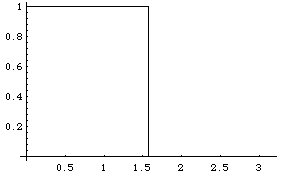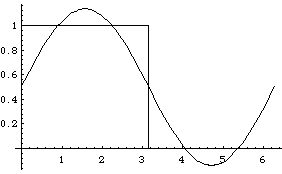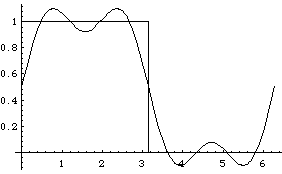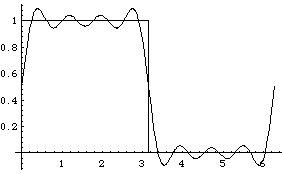# Orthogonal series and boundary value problems Calculations of some Fourier series

This is an evaluated Mathematica notebook. If you have Mathematica or MathReader (which is available free from WRI, you may download the notebook file. This notebook contains some Mathematica calculations for chapter IV, The Geometry of Functions, of Harrell's WWW textbook.

Let's evaluate the Fourier series for the functions:
f(x) = 1 for 0 <= x <= L/2, but 0 for L/2 <= x <= L.
and
g(x) = x, 0 <= x <= L.

We want to represent these functions in the form
a + Sum[a[m] Cos[2 Pi m x/L, {m,1,N}] + Sum[b[n] Sin[2 Pi n x/L, {n,1,N}]. By
the way, there is a procedure in Mathematica called Fourier, but it computes the discrete
Fourier transform, which is a related but different operation.

The formulae for these coefficients are:

a := (1/L) Integrate[f[x], {x,0,L}] (the average of f)
a[m] := (2/L) Integrate[f[x] Cos[2 Pi m x/L], {x,0,L}], m = 1, 2, ...
b[n] := (2/L) Integrate[f[x] Cos[2 Pi n x/L], {x,0,L}], n = 1, 2, ...

In:=

```  f[x_,L_] := 1 /; 0 <= x <= L/2
f[x_,L_] := 0 /; L/2 < x <= L```

In:=

`  Plot[f[x,Pi], {x, 0, Pi}]`

Out=```-Graphics-
```

In:=

`  a := (1/L) Integrate[f[x,L], {x,0,L}]`

In:=

`  a`

Out=

```  1
-
2
```

When calculating the Fourier coefficients with Mathematica, it is often useful to inform Mathematica of some simplifications of the trig functions, with a command such as

```
In:=  TrigId = {Cos[Pi n_] -> (-1)^n, Sin[Pi n_] -> 0}
Out:=  {Cos[Pi*(n_)] -> (-1)^n, Sin[Pi*(n_)] -> 0}

```
This tells the software to replace Cos[Pi n] with (-1)^n wherever it is encountered. (If n were not an integer, this would not be true.)

In:=

`  TrigId = {Cos[Pi n_] -> (-1)^n, Sin[Pi n_] -> 0}`

Out=

```                       n
{Cos[Pi (n_)] -> (-1) , Sin[Pi (n_)] -> 0}
```

In:=

`  a[m_,L_] := (2/L) Integrate[Cos[2 m Pi x/L], {x,0,L/2}]`

In:=

`  a[m,Pi]`

Out=

```  Sin[m Pi]
---------
m Pi
```

In:=

`  % /. TrigId`

Out=

```  0
```

These coefficients are all zero. Why?

In:=

`  b[n_,L_] := (2/L) Integrate[Sin[2 n Pi x/L], {x,0,L/2}]`

In:=

`  b[n,Pi] /. TrigId`

Out=

```               n
1    (-1)
2 (--- - -----)
2 n    2 n
---------------
Pi
```

In:=

`  Simplify[%]`

Out=

```          n
1 - (-1)
---------
n Pi
```

If n is even, b[2 k] = 0. Thus only odd terms survive, in which case
b[2 k + 1] = 2/(Pi (2 k + 1)). We can write the full series:

In:=

`  Clear[FullSeries]`

In:=

```  FullSeries[x_,N_,L_] := 1/2 + \
Sum[2 Sin[2 Pi n x/L]/ (Pi n),{n,1,N,2}] ```

In:=

`  Plot[{FullSeries[x,1, 2 Pi], f[x,2 Pi]}, {x,0,2 Pi}]`

Out=```-Graphics-
```

In:=

`  Plot[{FullSeries[x,3, 2 Pi], f[x,2 Pi]}, {x,0,2 Pi}]`

Out=```  -Graphics-
```

In:=

`  Plot[{FullSeries[x,7, 2 Pi], f[x,2 Pi]}, {x,0,2 Pi}]`

Out=```  -Graphics-
```

We can now investigate some questions about the excitation of mechanical resonances.
Suppose that experiments by K. Battle at the Wiener Staa